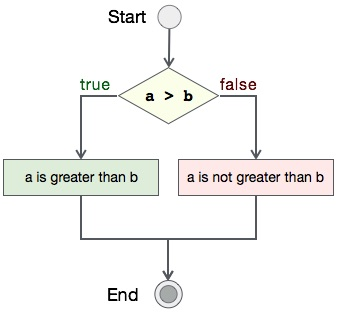# Counting program in C

Comparing two integer variables is one of the simplest program you can write at ease. In this program, you can either take input from user using `scanf()` function or statically define in the program itself.

We expect it to be a simple program for you as well. We are just comparing two integer variables. We shall first look the algorithm, then its flow diagram followed by pseudocode and implementation.

## Algorithm

Let's first see what should be the step-by-step procedure to compare two integers−

```START

Step 1 → Take two integer variables, say A & B

Step 2 → Assign values to variables

Step 3 → Compare variables if A is greater than B

Step 4 → If true print A is greater than B

Step 5 → If false print A is not greater than B

STOP
```

## Flow Diagram

We can draw a flow diagram for this program as given below −## Pseudocode

Let's now see the pseudocode of this algorithm −

```procedure compare(A, B)

IF A is greater than B
DISPLAY "A is greater than B"
ELSE
DISPLAY "A is not greater than B"
END IF

end procedure
```

## Implementation

Now, we shall see the actual implementation of the program −

```#include <stdio.h>

int main()
{
int i;

for(i = 1; i <= 10; i++)
printf("%2d\n", i);

return 0;
}
```

## Output

Output of this program should be −

``` 1
2
3
4
5
6
7
8
9
10
```